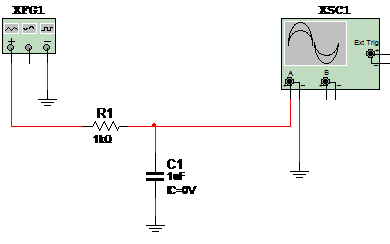### DatasheetsN° of component

## Circuit for Capacitor Test (MSB074E)

This simulation shows how can we test a capacitor using a function generator and oscilloscope. The simulation applies a 1 kHz x 10 Vpp to a capacitor under test and shows the wave shape in the RC circuit. The resistor R with the capacitor under test form a differentiator circuit and the oscilloscope shows the wave shape. The circuit is shown in figure 1.Figure 1 – The circuit

The wave shape in the virtual oscilloscope of the Multisim is shown in figure 2.Figure 2 – Wave shape in the circuit

Download the simulation files and Netlist - click here (msb0074.zip)